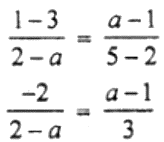Newbie

# Find the value of ‘a’ for which the following points A (a, 3), B (2,1) and C (5, a) are collinear. Hence find the equation of the line.

• 1

M.L Aggarwal book Important Question of class 10 chapter Based on Equation of a Straight Line for ICSE BOARD.
Here some points are given that are collinear
A (a, 3), B (2,1) and C (5, a)
You have to find the value of a and the equation of the line.
This is the Question Number 20 Exercise 12.1 of M.L Aggarwal.

Share

1.Given,

Points A (a, 3), B (2,1) and C (5, a) are collinear.

So, slope of AB = slope of BC⇒ -6 = (a – 1) (2 – a) [On cross multiplying]

-6 = 2a – 2 – a2 + a

-6 = 3a – a2 – 2

a2 – 3a – 4 = 0

a2 – 4a + a – 4 = 0

a (a – 4) + (a – 4) = 0

(a + 1) (a – 4) = 0

a = -1 or 4

As a = -1 doesn’t satisfy the equation

⇒ a = 4

Now,

Slope of BC = (a – 1)/(5 – 2) = (4 – 1)/3 = 3/3 = 1 = m

So, the equation of BC is

(y – 1) = 1 (x – 2)

y – 1 = x – 2

x – y = -1 + 2

Thus, the equation of BC is x – y = 1.

• 1